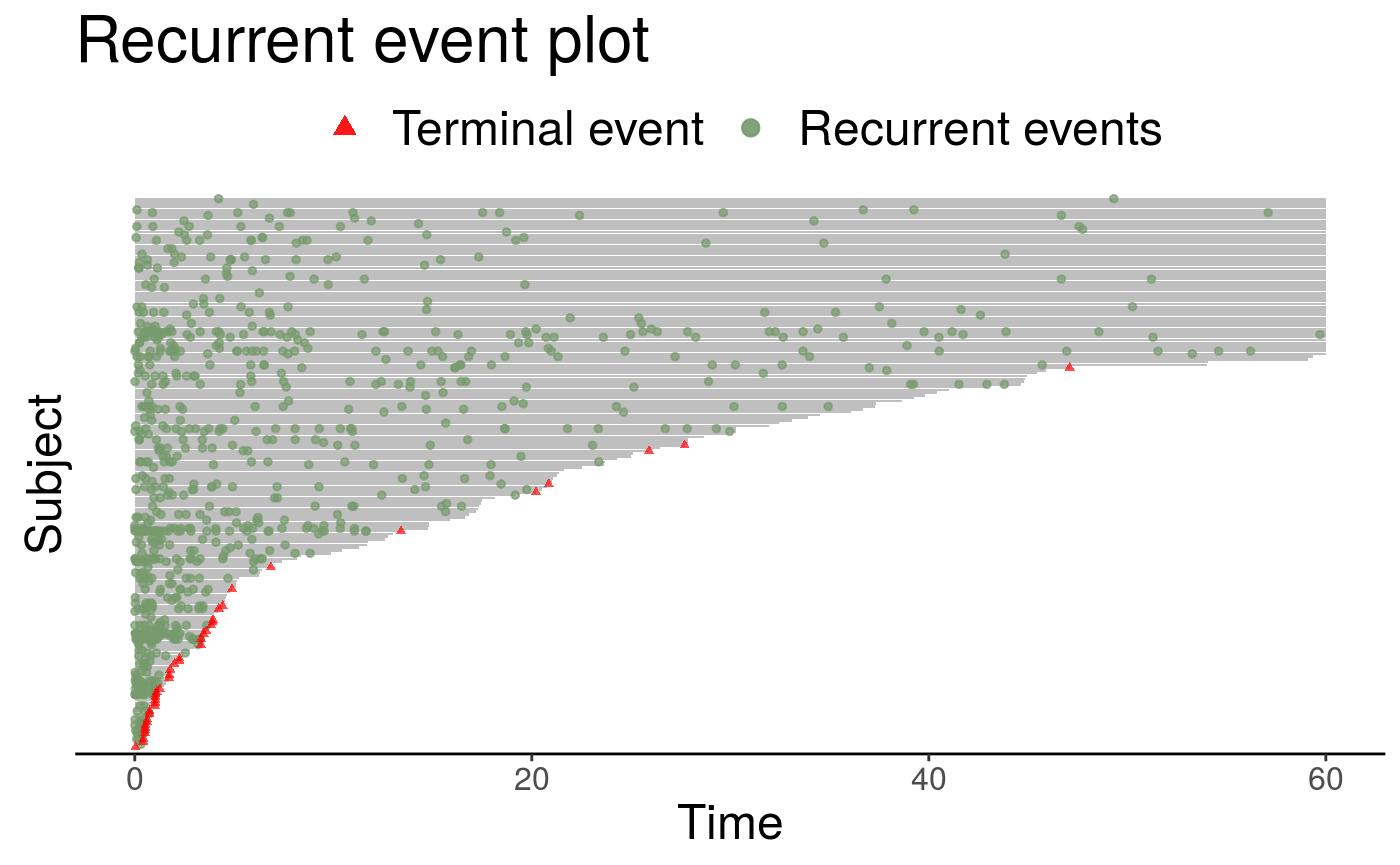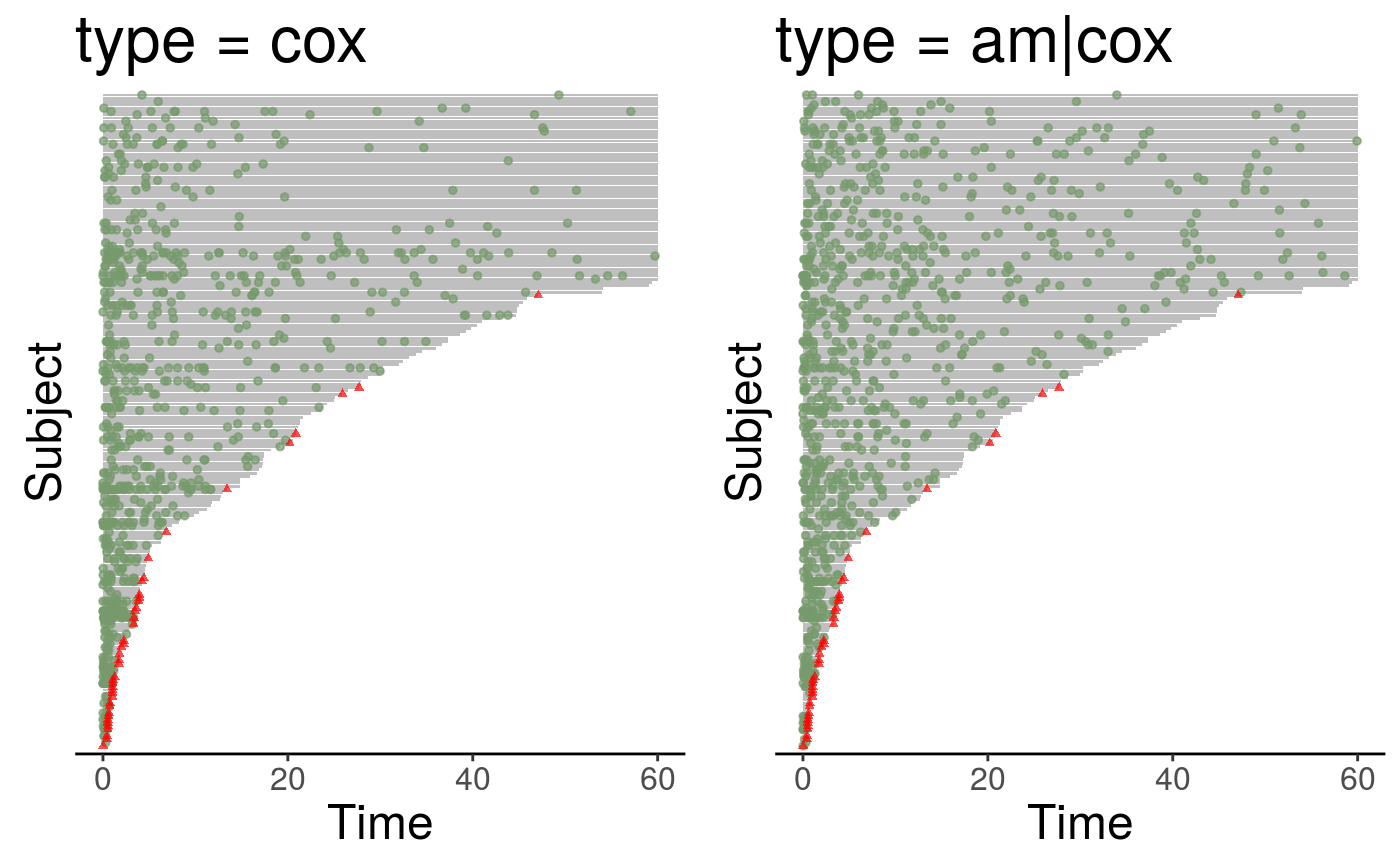In this vignette, we demonstrate how to use the simSC() function in reReg package to simulate recurrent event data from a scale-change model. Since the scale-change model includes the Cox-type model and the accelerated mean model as special cases, simSC() can also be used to generate data from these submodels. The simSC() function allows the censoring time to be non-informative (independent given covariate) or informative about the recurrent event process.

## Notations

Suppose recurrent events can potentially be observed in the time period $$[0, \tau]$$. For a subject, let $$N_i(t)$$ be the number of events in interval $$[0, t]$$, and $$X_i$$ is a $$p\times 1$$ covariate vector. Let $$C_i$$ be a non-informative censoring time, which is independent of $$N_i(\cdot)$$ given $$X_i$$. On the contrary, let $$D_i$$ be a failure time (informative censoring time), which is associated with $$N_i(\cdot)$$ even after conditioning on $$X$$. Then the follow-up time is defined as $$Y = \min(C, D, \tau)$$. The observed data are independent and identically distributed copies of $$\{N_i(t), Y_i, X_i: t\le Y_i, i = 1, \ldots, n\}$$. In the following, we suppress the index for the ease of discussion.

## The simSC function

The function simSC generates the recurrent times from a recurrent event process, $$N(t)$$, from a pre-specified rate function, $$\lambda(t)$$, and the failure time, $$D$$, from a pre-specified hazard function, $$h(t)$$. A general joint model for the rate function and the hazard function can be formulated in the following: \begin{equation} \begin{matrix} \lambda(t) &= Z\lambda_0(te^{X^\top\alpha})e^{X^\top\beta};\\ h(t) &= Zh_0(te^{X^\top\eta})e^{X^\top\theta}, \end{matrix} \label{eq:joint} \end{equation}

where $$Z$$ is a latent shared frailty variable, $$(\alpha, \eta)$$ and $$(\beta, \theta)$$ correspond to the shape and size parameters of the rate function and the hazard function, respectively. The frailty variable $$Z$$ is used to capture the association between the rate function and the hazard function, thus, accommodating informative censoring. The simSC() currently only allows two covariates, i.e., $$X = (X_{1}, X_{2})^\top$$, where $$X_1$$ is a Bernoulli random variable with probability 0.5 and $$X_2$$ is a standard normal random variable. The frailty variable $$Z$$ is generated from a gamma distribution with mean 1. The non-informative censoring time, $$C$$, is generated separately from an exponential distribution with mean 80. The observed follow-up time is then taken to be $$Y = \min(D, C, \tau)$$. We further assume the baseline functions $\lambda_0(t) = \frac{2}{1 + t}, h_0(t) = \frac{1}{8(1 + t)}.$

The arguments in simSC are as follow

> library(reReg)
> args(simSC)
function (n, a1 = a2, b1 = b2, a2 = a1, b2 = b1, type = "cox",
zVar = 0.25, tau = 60, origin = 0, summary = FALSE)
NULL

The arguments are as follows

• n is the number of individual
• a1, a2, b1, b2 are numeric vectors of length two, these arguments corresponding to $$\alpha$$, $$\beta$$, $$\eta$$, and $$\theta$$ from \eqref{eq:joint}, respectively.
• type is a character string specifying the underlying model. The rate function type and the hazard function type are separated by a vertical bar |, with the rate function on the left. For example, type = "cox|am" generates the recurrent process from a Cox model and the terminal event from an accelerated mean model. Setting type = "cox" gives type = "cox|cox".
• zVar is a numeric value specifying the variance of the frailty variable, $$Z$$.
• tau is a numeric value specifying the maximum observation time, or $$\tau$$ in the above notation.
• summary is a logical value indicating whether a brief data summary will be printed.

## Examples

In the following examples, we simulate recurrent event data when both the rate function and the hazard function are Cox-type models and use summary = TRUE to display some descriptive statistics about the simulated data.

> set.seed(273)
> dat.cox <- simSC(200, c(-1, 1), c(-1, 1), summary = TRUE)

Summary results for number of recurrent event per subject:
Min. 1st Qu.  Median    Mean 3rd Qu.    Max.
0.0     1.0     3.0     4.1     5.0    34.0

Number of failures: 41 (20.5%); Number of censored events: 159 (79.5%)

Number of x1 == 1: 119 (59.5%); Number of x1 == 0: 81 (40.5%)
Summary results for x2:
Min.  1st Qu.   Median     Mean  3rd Qu.     Max.
-2.94095 -0.51231  0.03091  0.06854  0.70469  2.14260 

The output of simSC() is a data frame. The following table shows that subject #1 experienced 0 recurrent events (at times 17.467) and died at time 17.467. Similarly, subject #2 experienced 3 recurrent events (at times 3.676, 7.72, 53.272) and is alive when censored at time 60.

> library(DT)
> datatable(dat.cox, options = list(pageLength = 10, scrollX = TRUE)) %>%
+   formatRound(c("t.stop", "x2"), 3)

The event plot for the above simulated data is
> plotEvents(Recur(t.stop, id, event, status) ~ 1, data = dat.cox)With the same random seed, the following example generates recurrent event data when the rate function is an accelerated mean model while the hazard function is a Cox model. Compare to the previous example, the difference is in the model structure of the rate function.

> set.seed(273)
> dat.amcox <- simSC(200, c(-1, 1), c(-1, 1), type = "am|cox")
> datatable(dat.amcox, options = list(pageLength = 10, scrollX = TRUE)) %>%
+   formatRound(c("t.stop", "x2"), 3)

Here, subject #1 experienced 0 recurrent events (at times 17.467) and died at time 17.467. Similarly, subject #2 experienced 7 recurrent events (at times 2.282, 3.802, 12.045, 14.722, 16.946, 23.234, 41.396) and is alive when censored at time 60.

A side-by-side event plot shows the difference in the recurrent process.

> library(ggplot2)
> library(gridExtra)
> grid.arrange(plotEvents(Recur(t.stop, id, event, status) ~ 1,
+                         data = dat.cox,
+                         main = "type = cox") +
+              theme(legend.position = "none"),
+              plotEvents(Recur(t.stop, id, event, status) ~ 1,
+                         data = dat.amcox,
+                         main = "type = am|cox") +
+              theme(legend.position = "none"),
+              ncol = 2)